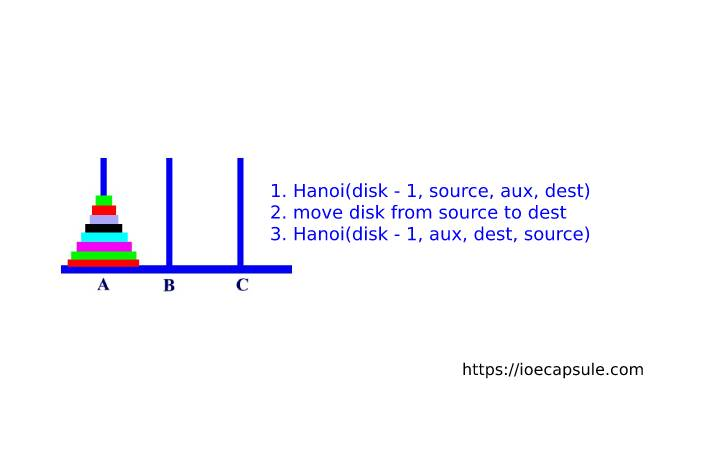### Tower Of Hanoi – Algorithm And ImplementationAlgorithms

Tower Of Hanoi or TOH in short, is a game containing three pegs A, B, C and A contains disks of different diameters in such a way that larger disk is always below a smaller disk. The objective is to move the disks from peg A to peg B, using peg C as auxiliary following following rules :-

1. Only one disk can be moved among the towers at any given time.
2. Only the “top” disk can be removed.
3. No large disk can sit over a small disk.

Following GIF image represents the solving of Tower Of Hanoi with three disks.

Tower of Hanoi puzzle with n disks can be solved in minimum 2n−1 steps. This presentation shows that a puzzle with 3 disks has taken 23 – 1 = 7 steps.

### Algorithm Of Tower Of Hanoi Puzzle

`A = source peg, B = destination peg, C = auxiliary peg`
1. If n = 1, move the single disk from A to B and stop.
2. Move the top n-1 disks from A to C(auxiliary peg).
3. Move the remaining nth disk from A to B.
4. Move the n-1 disk from C to B using A as auxiliary.

### Pseudo code of Tower Of Hanoi

``````
START
Procedure Hanoi(disk, source, dest, aux)
IF disk == 1, THEN
move disk from source to dest          // Step 1
ELSE
Hanoi(disk - 1, source, aux, dest)     // Step 2
move disk from source to dest          // Step 3
Hanoi(disk - 1, aux, dest, source)     // Step 4
END IF
END Procedure
STOP
``````

### C function to solve Tower Of Hanoi Puzzle

``````
// C function to solve tower of hanoi puzzle recursively
void towerOfHanoi(int n, char from, char to, char aux)
{
if (n == 1)
{
printf("\n Move disk 1 from rod %c to rod %c", from, to);
return;
}
towerOfHanoi(n-1, from, aux, to);
printf("\n Move disk %d from rod %c to rod %c", n, from, to);
towerOfHanoi(n-1, aux, to, from);
}
``````